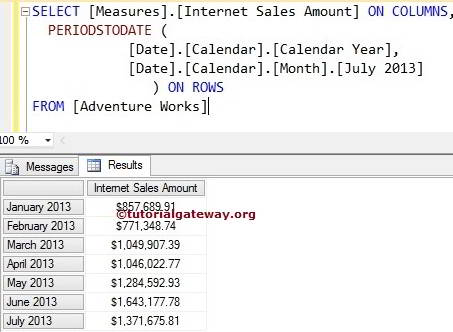# MDX PeriodsToDate Function

The MDX PeriodsToDate Function used to navigate between same level members. It returns the set of sibling members from First Sibling to the specified member. For example, If you want to list the members from one position to a particular member, then we can use this MDX PeriodsToDate function.

## MDX PeriodsToDate Function Syntax

The basic syntax of the MDX PeriodsToDate in Multidimensional Expression is as shown below:

`PERIODSTODATE (Level_Expression, Member_Expression)`
• Member_Expression: Any Multidimensional Expression that returns valid Member.
• Member_Position: Please specify the position of a member you want to Navigate.

MDX PeriodsToDate Function used to navigate between the same Members level. For example, If we mentioned City member as Member_Expression, then PeriodsToDate function will navigate between remaining Cities. It will not go Level Up (State – Province) or Level down (Postal Code). For this MDX PeriodsToDate Function example, we are going to use below shown data.

## MDX PeriodsToDate Function Example 1

In this example, we will show you, What happens when we use Zero value for the PeriodsToDate Function. The following query will return the Internet Sales Amount of Months present in Calendar Year 2013 until it reaches July 2013

```SELECT
[Measures].[Internet Sales Amount] ON COLUMNS,
PERIODSTODATE (
[Date].[Calendar].[Calendar Year],
[Date].[Calendar].[Month].[July 2013]
) ON ROWS
```In the above MDX Query, We used [Reseller Sales amount] on the columns

`[Measures].[Reseller Sales Amount] ON COLUMNS`

Below line of code will check

```PERIODSTODATE (
[Date].[Calendar].[Calendar Year],
[Date].[Calendar].[Month].[July 2013]
)```

for the France parent (Which is All member) and then finds the Last child member of the Country.

## MDX Periods To Date Function Example 2

In this example, we will show you, What happens when we use Positive integer value in PeriodsToDate Function. The following query will return the Internet Sales amount of the Calendar Year 2013, 2012, and 2011 because LastPeriods function with Positive value (3) will move back 2 years + year 2013. It is because the PeriodsToDate function includes 2013 itself.

```SELECT
[Measures].[Internet Sales Amount] ON COLUMNS,
PERIODSTODATE (
[Date].[Calendar].[(All)],
[Date].[Calendar].[Calendar Year].[CY 2013]
) ON ROWS